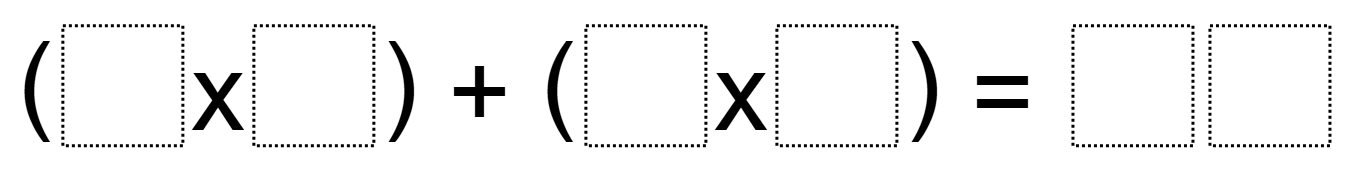Home > Grade 4 > Adding Multiples

Directions: Using the digits 0 to 9, at most one time each, fill in the boxes to make a true statement.### Hint

How can you choose digits for your addends so that the sum is a double digit number?

There are multiple answers including:
(6 x 4) + (3 x 9) = 51
(8 x 1) + (4 x 3) = 20

Source: Owen Kaplinsky

## Multiplying Differences

Directions: Using the digits 1 to 9, at most one time each, fill in the …

### One comment

1.wouldn’t 8 times 7 plus 2 times 10 equals 76 me true